# Geometric Integration of Differential Equations 1 Introduction and

• Slides: 31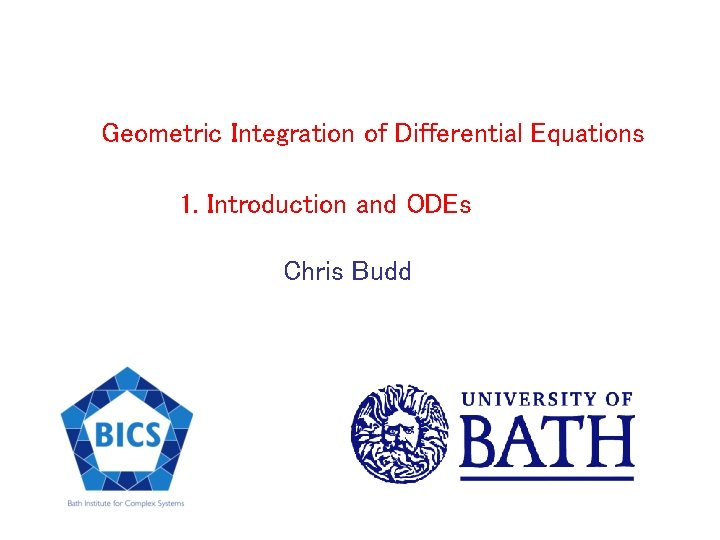Geometric Integration of Differential Equations 1. Introduction and ODEs Chris BuddWant to simulate a physical system governed by differential equations Expect the numerical approximation to have the same qualitative features as the underlying solution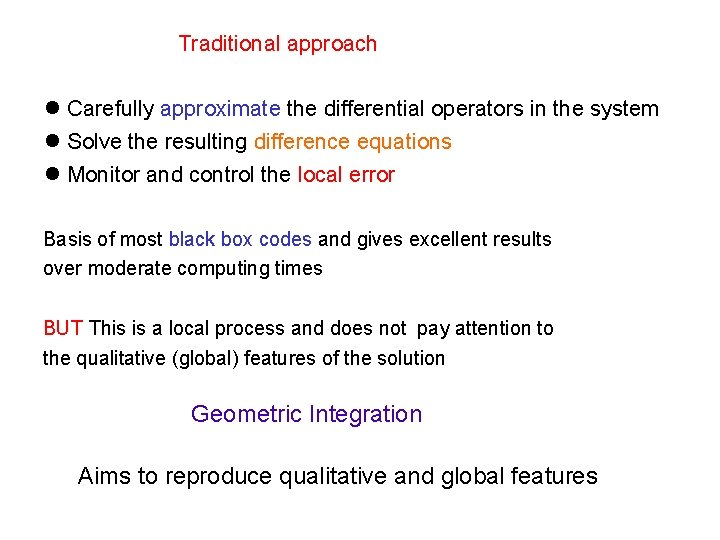Traditional approach Carefully approximate the differential operators in the system Solve the resulting difference equations Monitor and control the local error Basis of most black box codes and gives excellent results over moderate computing times BUT This is a local process and does not pay attention to the qualitative (global) features of the solution Geometric Integration Aims to reproduce qualitative and global features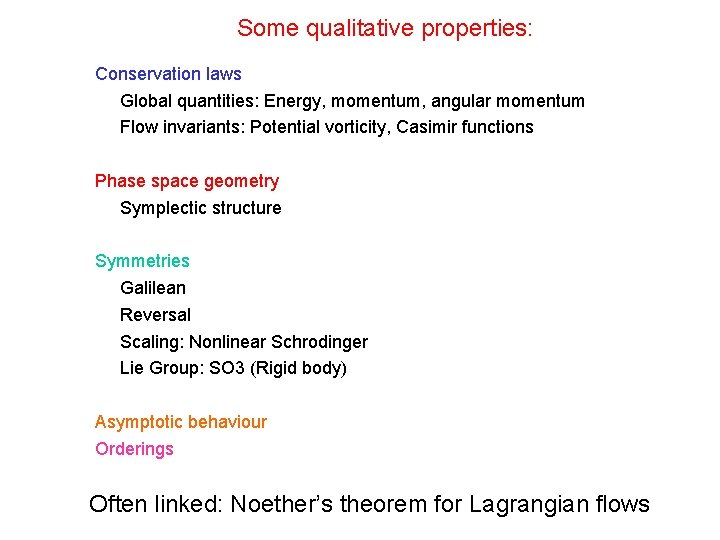Some qualitative properties: Conservation laws Global quantities: Energy, momentum, angular momentum Flow invariants: Potential vorticity, Casimir functions Phase space geometry Symplectic structure Symmetries Galilean Reversal Scaling: Nonlinear Schrodinger Lie Group: SO 3 (Rigid body) Some global features Asymptotic behaviour Orderings Often linked: Noether’s theorem for Lagrangian flowsExample: The Kepler Problem Conserved quantities: Hamiltonian Angular Momentum Symmetries: Rotation, Reflexion, Time reversal, Scaling Kepler's Third LawGeometric Integration Aims to preserve a subset of these features Take advantage of powerful global error estimates (shadowing) Powerful methods for important physical problems Examples of GI methods Symplectic and multi-symplectic Splitting Lie Group/Magnus Discrete Lagrangian Scale Invariant Examples of GI applications Molecular and celestial mechanics Rigid body mechanics Weather forecasting Integrable systems (optics) Self-similar PDEs Highly oscillatory problemsExample of the traditional and the GI approach: Integrating the Harmonic Oscillator Qualitative features: Bounded periodic solutions, time reversal symmetry, Conserved Forward Euler method (non GI)Problem: Energy increases, lack of periodicity, lack of symmetry Backward Euler Method (non GI) Problem: Energy decreases, lack of periodicity, lack of symmetry Mid-point rule (a GI method)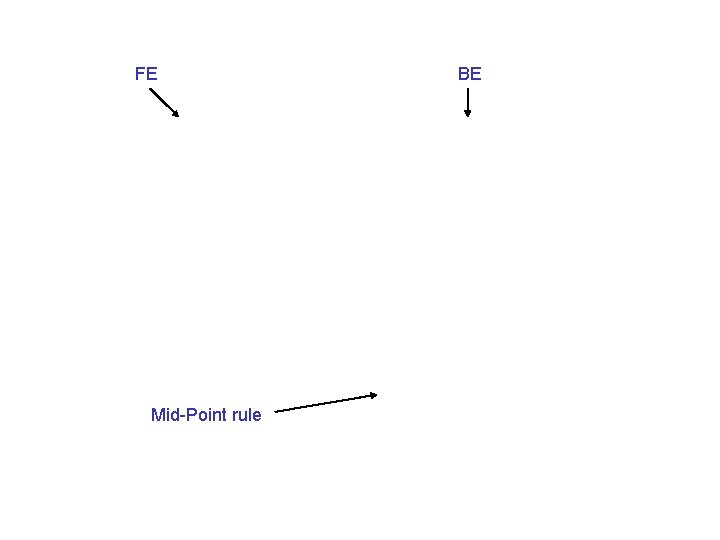FE Mid-Point rule BEMid point rule conserves: Energy Symmetry Backward (Modified) Equation Analysis Discrete equation has an exact solution Solutions are: Bounded, periodic Phase error proportional to Discrete solution shadows the continuous one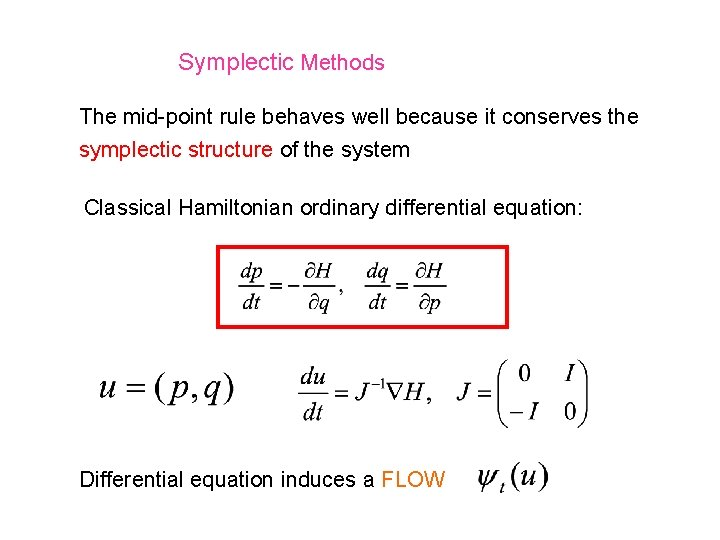Symplectic Methods The mid-point rule behaves well because it conserves the symplectic structure of the system Classical Hamiltonian ordinary differential equation: Differential equation induces a FLOW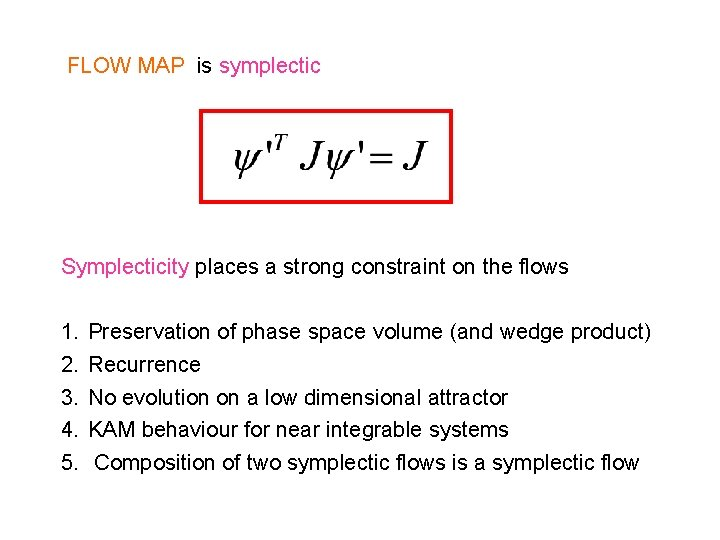FLOW MAP is symplectic Symplecticity places a strong constraint on the flows 1. 2. 3. 4. 5. Preservation of phase space volume (and wedge product) Recurrence No evolution on a low dimensional attractor KAM behaviour for near integrable systems Composition of two symplectic flows is a symplectic flow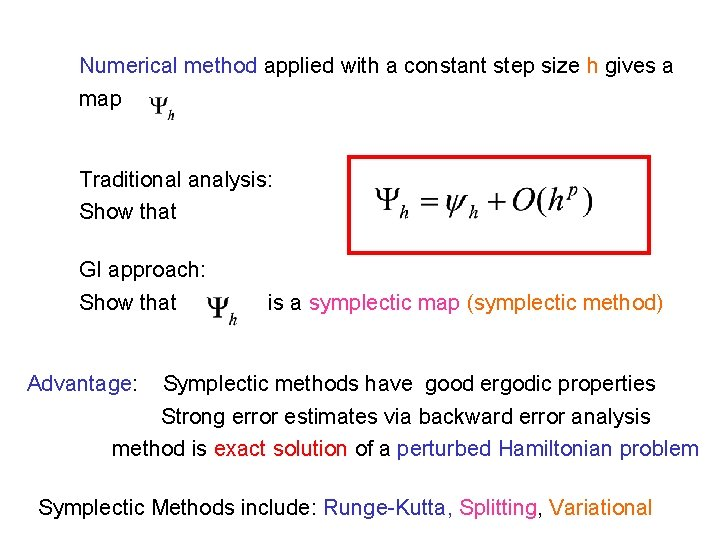Numerical method applied with a constant step size h gives a map Traditional analysis: Show that GI approach: Show that is a symplectic map (symplectic method) Advantage: Symplectic methods have good ergodic properties Strong error estimates via backward error analysis method is exact solution of a perturbed Hamiltonian problem Symplectic Methods include: Runge-Kutta, Splitting, VariationalRunge-Kutta methods for du/dt = f(u) There is a large class of implicit symplectic Runge-Kutta methods Butcher Tableaux c A b Construct matrix M Method is symplectic if M = 0 All linear and quadratic invariants conservedExample: the implicit mid-point rule Symplectic, implicit, symmetric, unconditionally stable, Conserves linear and quadratic invariants All Gauss-Legendre Runge-Kutta methods and associated collocation methods are symplectic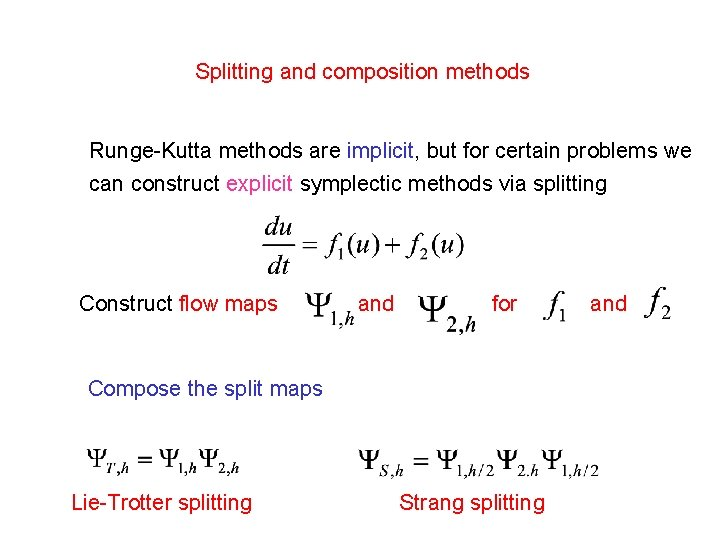Splitting and composition methods Runge-Kutta methods are implicit, but for certain problems we can construct explicit symplectic methods via splitting Construct flow maps and for Compose the split maps Lie-Trotter splitting Strang splitting andSome important results If and are symplectic, so are The Campbell-Baker-Hausdorff theorem implies that If H(u) = T(p) + V(q) The splittings lead directly to two important numerical methods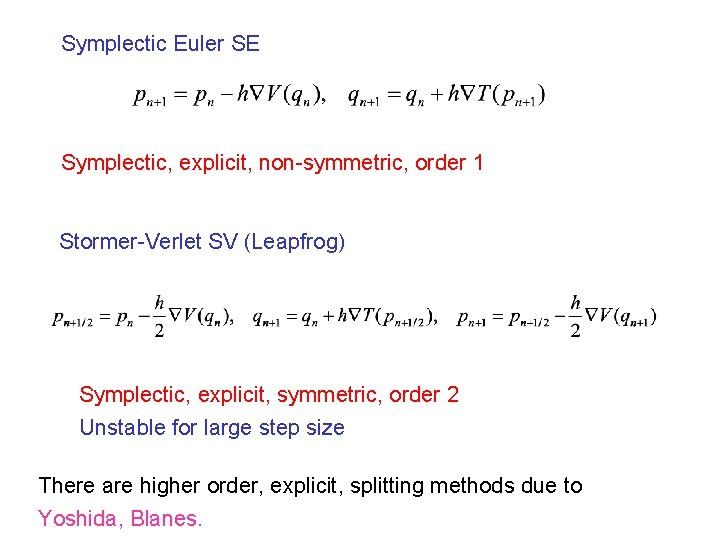Symplectic Euler SE Symplectic, explicit, non-symmetric, order 1 Stormer-Verlet SV (Leapfrog) Symplectic, explicit, symmetric, order 2 Unstable for large step size There are higher order, explicit, splitting methods due to Yoshida, Blanes.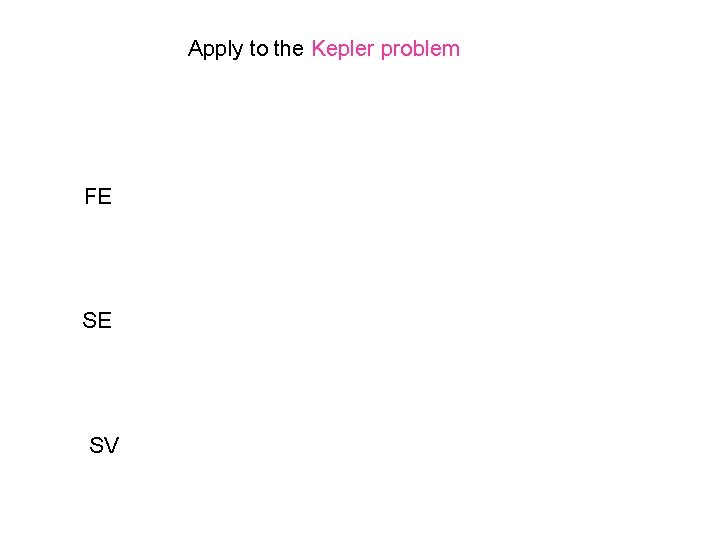Apply to the Kepler problem FE SE SV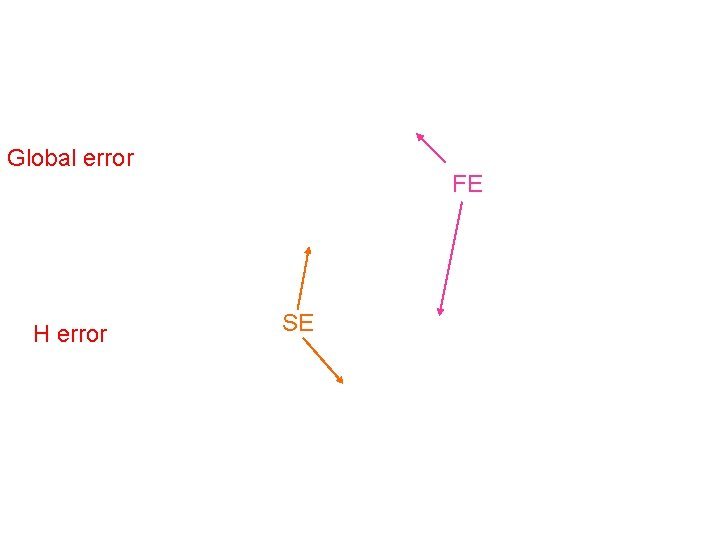Global error H error FE SEMethod FE SE SV Global error H error L error t^2 h th t h^2 th h h^2 th 0 0 NOTE: Kepler’s third law is NOT conserved by these methods … see the next talk!Backward Error Analysis Up to an exponentially small (In h) error the solutions of a symplectic method of order p are the discrete samples of a solution of a related Hamiltonian differential equation with Hamiltonian Can construct the perturbed Hamiltonian explicitly H error remains bounded for all times Doesn’t apply if h varies!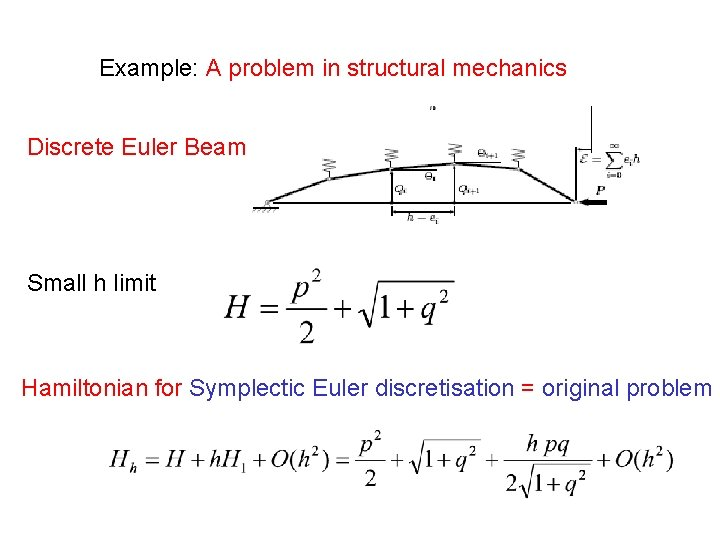Example: A problem in structural mechanics Discrete Euler Beam Small h limit Hamiltonian for Symplectic Euler discretisation = original problem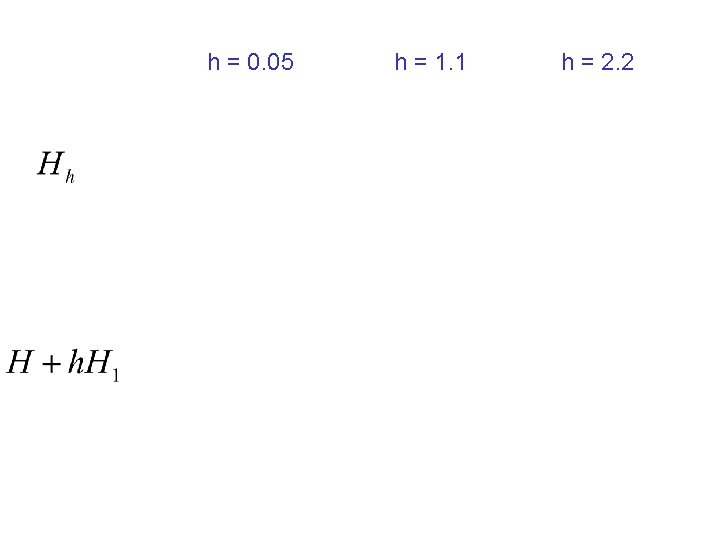h = 0. 05 h = 1. 1 h = 2. 2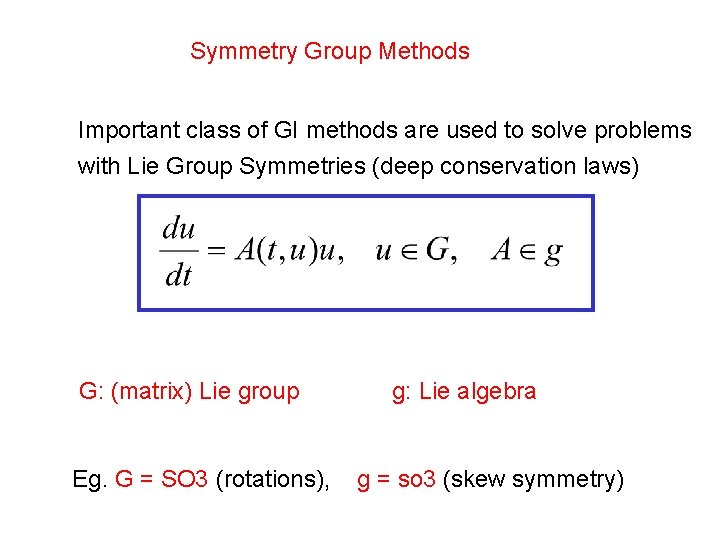Symmetry Group Methods Spo Important class of GI methods are used to solve problems tty with Lie Group Symmetries (deep conservation laws) dog G: (matrix) Lie group Eg. G = SO 3 (rotations), g: Lie algebra g = so 3 (skew symmetry)Can a numerical method ensure that the solution remains in G? Rigid body mechanics, weather forecasting, quantum mechanics, Lyapunov exponents, QR factorisation Idea: Do all computations in the Lie Algebra (linear space) And map between this and the Lie Group (nonlinear space) G numerical method gExamples of maps from g to G General g , G g = so 3, G = SO 3 satisfies the dexpinv equation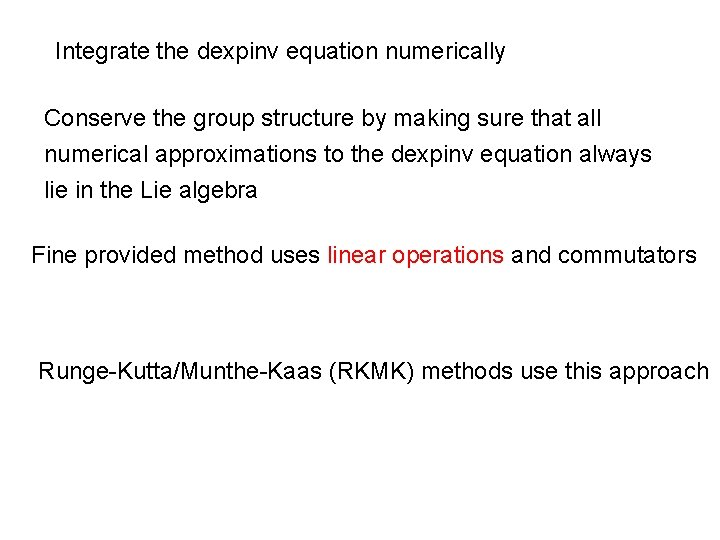Integrate the dexpinv equation numerically Conserve the group structure by making sure that all numerical approximations to the dexpinv equation always lie in the Lie algebra Fine provided method uses linear operations and commutators Runge-Kutta/Munthe-Kaas (RKMK) methods use this approach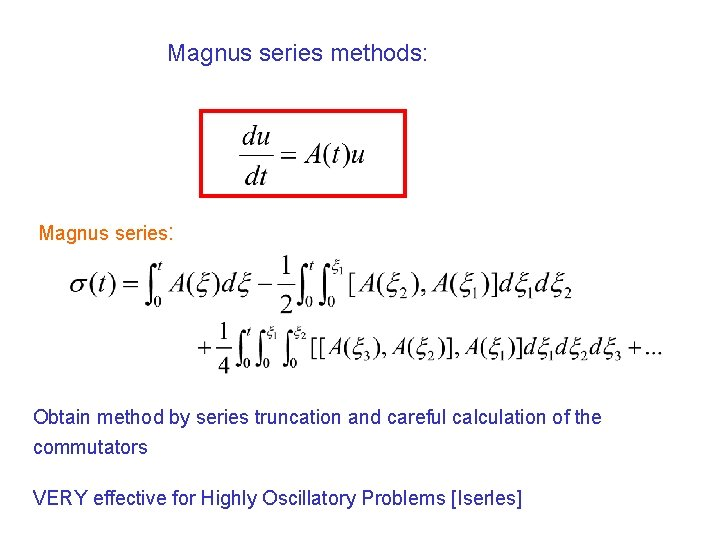Magnus series methods: Magnus series: Obtain method by series truncation and careful calculation of the commutators VERY effective for Highly Oscillatory Problems [Iserles]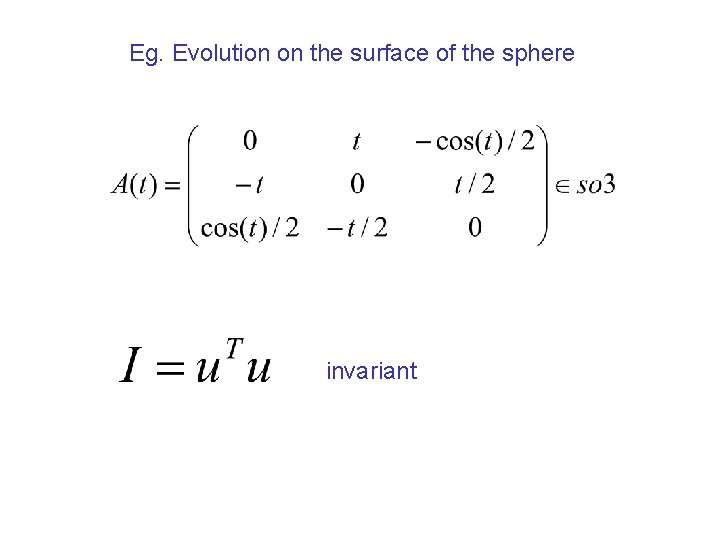Eg. Evolution on the surface of the sphere invariant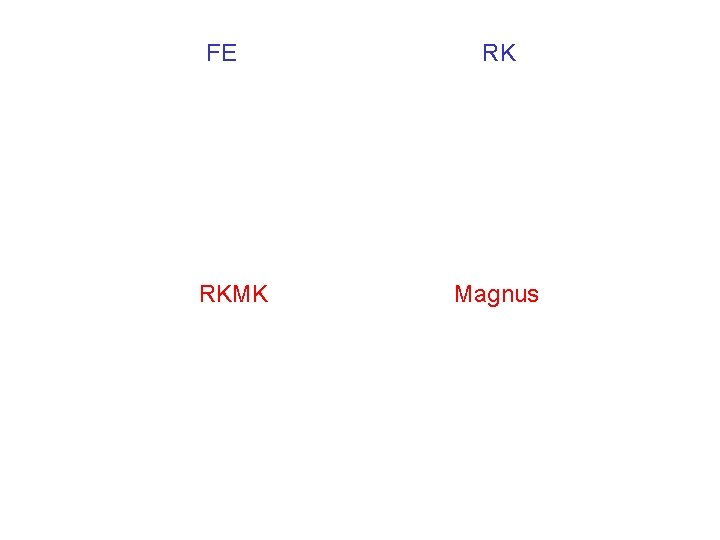FE RKMK RK Magnus